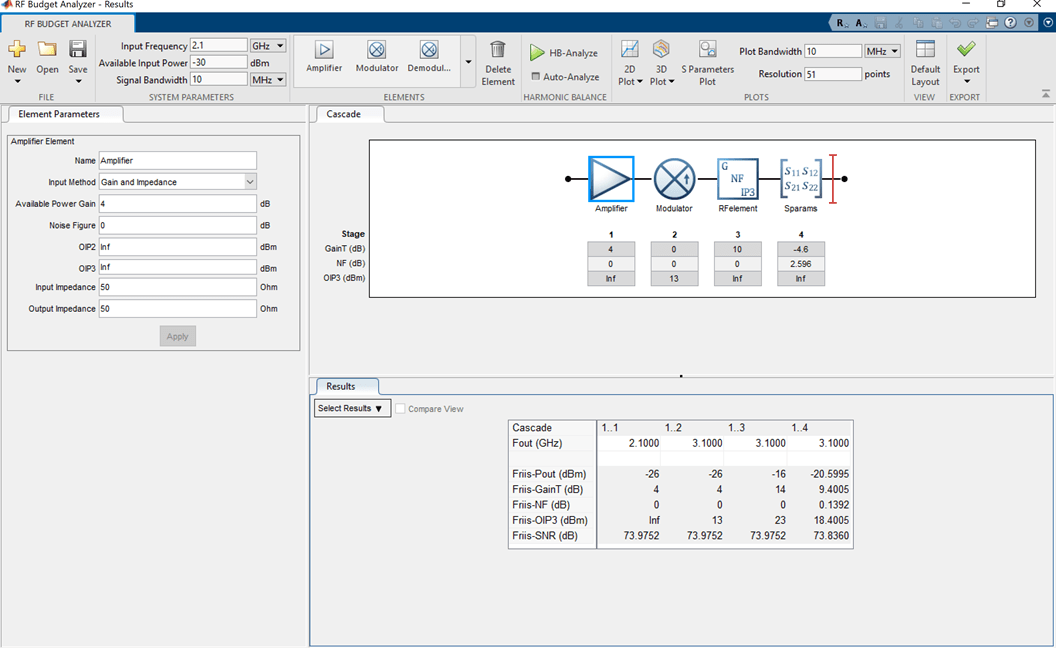amplifier

Amplifier object

Description

Use the amplifier object to create an amplifier element. An amplifier is a 2-port RF circuit object. You can use this element in the rfbudget object and the circuit object.

Creation

Description

example

amp = amplifier creates an amplifier object with default property values.

example

amp = amplifier(Name,Value) sets properties using one or more name-value pairs. You can specify multiple name-value pairs. Enclose each property name in a quote.

Properties

expand all

Name of amplifier, specified as a character vector. All names must be valid MATLAB® variable names.

Example: 'Name','amp'

Example: amplifier.Name = 'amp'

Available power gain, specified as a real finite scalar in dB.

Example: 'Gain',10

Example: amplifier.Gain = 10

Noise figure, specified as a real finite nonnegative scalar dB.

Example: 'NF',-10

Example: amplifier.NF = -10

Second -order output-referred intercept point, specified as a real scalar in dBm.

Example: 'OIP2',8

Example: amplifier.OIP2 = 8

Third -order output-referred intercept point, specified as a real scalar in dBm.

Example: 'OIP3',10

Example: amplifier.OIP3 = 10

Input impedance, specified as a positive real part finite scalar in ohms. You can also use a complex value with a positive real part.

Example: 'Zin',40

Example: amplifier.Zin = 40

Output impedance, specified as a scalar in ohms. You can also use a complex value with a positive real part.

Example: 'Zout',40

Example: amplifier.Zout = 40

Number of ports, specified as a scalar integer. This property is read-only.

Names of port terminals, specified as a cell vector. This property is read-only.

Object Functions

 clone Create copy of existing circuit element or circuit object

Examples

collapse all

Create an amplifier object named 'LNA' and has a gain of 10 dB.

a = amplifier('Name','LNA','Gain',10)
a =
amplifier: Amplifier element

Name: 'LNA'
Gain: 10
NF: 0
OIP2: Inf
OIP3: Inf
Zin: 50
Zout: 50
NumPorts: 2
Terminals: {'p1+'  'p2+'  'p1-'  'p2-'}

Create an amplifier object with a gain of 4 dB. Create another amplifier object that has an output third-order intercept (OIP3) 13 dBm.

amp1 = amplifier('Gain',4);
amp2 = amplifier('OIP3',13);

Build a 2-port circuit using the amplifiers.

c = circuit([amp1 amp2])
c =
circuit: Circuit element

ElementNames: {'Amplifier'  'Amplifier_1'}
Elements: [1x2 amplifier]
Nodes: [0 1 2 3]
Name: 'unnamed'
NumPorts: 2
Terminals: {'p1+'  'p2+'  'p1-'  'p2-'}

Create an amplifier with a gain of 4 dB.

a = amplifier('Gain',4);

Create a modulator with an OIP3 of 13 dBm.

m = modulator('OIP3',13);

Create an nport using passive.s2p.

n = nport('passive.s2p');

Create an rf element with a gain of 10 dB.

r = rfelement('Gain',10);

Calculate the rf budget of a series of rf elements at an input frequency of 2.1 GHz, an available input power of -30 dBm, and a bandwidth of 10 MHz.

b = rfbudget([a m r n],2.1e9,-30,10e6)
b =
rfbudget with properties:

Elements: [1x4 rf.internal.rfbudget.Element]
InputFrequency: 2.1 GHz
AvailableInputPower: -30 dBm
SignalBandwidth:  10 MHz
Solver: Friis
AutoUpdate: true

Analysis Results
OutputFrequency: (GHz) [  2.1    3.1    3.1     3.1]
OutputPower: (dBm) [  -26    -26    -16   -20.6]
TransducerGain: (dB)  [    4      4     14     9.4]
NF: (dB)  [    0      0      0  0.1392]
IIP2: (dBm) []
OIP2: (dBm) []
IIP3: (dBm) [  Inf      9      9       9]
OIP3: (dBm) [  Inf     13     23    18.4]
SNR: (dB)  [73.98  73.98  73.98   73.84]

Show the analysis in the RF Budget Analyzer app.

show(b)